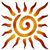# Arcade scripting in Attribute Rules

2123
19
04-01-2021 11:15 AM
Labels (2)Occasional Contributor II

Hi All,

I've gotten over my hurtle with creating attribute rules now, but I'm a newbie with Arcade - either in the WAB or in Pro.

I'm attempting to create a Calculation rule that is based on If Then Else statements.  I am getting a message about Invalid expression.  Error on line 8.  Open parenthesis expected.

Here's the beginning of my expression; at the end I do have return eru;  As this is the field that I am attempting to calculate.  There will be three of these set up so as to do the required pre-calcs before getting the last two calculations that are the real ones to be returned and saved.

It is highly likely that I'm missing just something simple, I thought that I was following the examples that I found for doing calcs and using if statements.  But ....

var fs = FeatureSetByName(\$datastore,"IDF_Stormwater");
var billclass = fs["MultiUserType"];
var eru = fs["ERUArea"];
var parea = fs["IDFArea"];
var pimparea = fs["ImperviousArea"];
var dwell = fs["Dwellings"];

if billclass == 'D'
eru = 0
else if billclass in ('V','I','H')
eru = Round(parea/43560,2)
else if billclass == 'R'
eru = Round(parea*(2000/7760)/2000,2)
else if billclass in ('A','O')

.....

Thanks,

Lorinda

Tags (3)
19 RepliesbyMVP Regular Contributor

You're missing a statement after the last.else if.Occasional Contributor II

Not really, the remainder of the script is after a few more lines:

else if billclass = 'R' && pimparea = 0
eru = Round(parea*(2000/7760)/2000,2)
else if billclass = 'R' && pimarea > 0
eru = (Round(pimparea/2000,2)

return eru;byMVP Esteemed Contributor

``````else if billclass = 'R' && pimparea = 0  /* Could this be a problem */
eru = Round(parea*(2000/7760)/2000,2)
else if billclass = 'R' && pimarea > 0   /* as well as this */
eru = (Round(pimparea/2000,2)

return eru;

/*
else if billclass == ('R' && pimparea = 0)
...
else if billclass == ('R' && pimarea > 0)
*/``````

That should just about do it....byMVP Regular Contributor

@LorindaGilbert wrote:

Not really, the remainder of the script is after a few more lines:

Which is where the error is.byMVP Esteemed Contributor
``````var fs = FeatureSetByName(\$datastore,"IDF_Stormwater");
var billclass = fs["MultiUserType"];
var eru = fs["ERUArea"];
var parea = fs["IDFArea"];
var pimparea = fs["ImperviousArea"];
var dwell = fs["Dwellings"];

if billclass == 'D'
eru = 0
else if billclass in ('V','I','H')
eru = Round(parea/43560,2)
else if billclass == 'R'
eru = Round(parea*(2000/7760)/2000,2)
else if billclass in ('A','O')
//// Need something here as Luke points out``````

I took the liberty to copy and paste your code into the code editor; makes it easier to read.  If you expand the three dots to expand the tool bar and then choose the  "Insert/Edit  Code Sample" tool that looks like this: </> you can format your code as it is meant to be read.  I used JavaScript for this...

That should just about do it....Occasional Contributor II

My full script is this:

var fs = FeatureSetByName(\$datastore,"IDF_Stormwater");
var billclass = fs["MultiUserType"];
var eru = fs["ERUArea"];
var parea = fs["IDFArea"];
var pimparea = fs["ImperviousArea"];
var dwell = fs["Dwellings"];

if billclass == 'D'
eru = 0
else if billclass in ('V','I','H')
eru = Round(parea/43560,2)
else if billclass == 'R'
eru = Round(parea*(2000/7760)/2000,2)
else if billclass in ('A','O')
eru = Round(pimparea/2000,2)
else if billclass == 'M' && dwell in (1,2)
imparea = parea*0.3192
else if bill class == 'M' && dwell in (3,4)
imparea = parea*0.5093
else if billclass == 'M' && dwell > 4
imparea = parea*0.5613
else if billclass in ('M','A','O') && pimparea = 0
eru = Round(pimpara/2000,2)
else if billclass = 'R' && pimparea = 0
eru = Round(parea*(2000/7760)/2000,2)
else if billclass = 'R' && pimarea > 0
eru = (Round(pimparea/2000,2)

return eru;

Yes, I see that I am missing an end if it is needed.byMVP Regular Contributor

eru = (Round(pimparea/2000,2)

return eru;

Either remove the bracket before Round or add a closing bracket.byMVP Esteemed Contributor

Good Catch @Luke_Pinner !

That should just about do it....Occasional Contributor II

Alas, that didn't solve the expected open parenthesis error.

Haven't checked what Joe Borgione mentioned with the two items on one line yet and the &&.  Had to start on another project in FME right now.  Will try this again tomorrow.